Next: 6. Limits of Sequences Up: 5. Area Previous: 5.5 Brouncker's Formula For   Index

# 5.6 Computer Calculation of Area

In this section we will discuss a Maple program for calculating approximate values of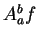for monotonic functions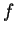on the interval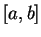. The programs will be based on formulas discussed in theorem 5.40.

Letbe a decreasing function from the intervalto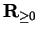, and let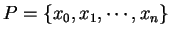be a partition of. We know that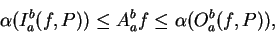where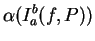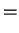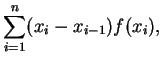(5.83)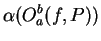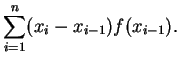(5.84)

Let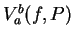be the average of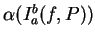and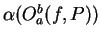, so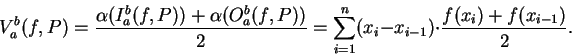Now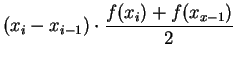represents the area of the trapezoid with vertices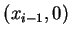,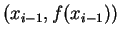,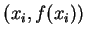and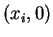, sorepresents the area under the polygonal line obtained by joining the pointsandfor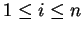.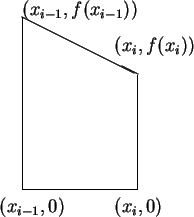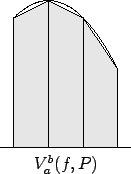In the programs below, leftsum(f,a,b,n) calculates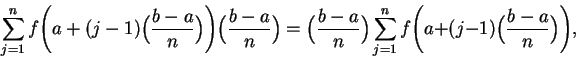which corresponds to (5.84) when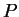is the regular partition ofinto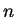equal subintervals, and rightsum(f,a,b,n) calculates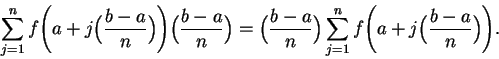which similarly corresponds to (5.83). The command average(f,a,b,n) calculates the average of leftsum(f,a,b,n) and rightsum(f,a,b,n).

The equation of the unit circle is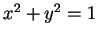, so the upper unit semicircle is the graph ofwhere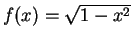. The area of the unit circle is 4 times the area of the portion of the circle in the first quadrant, so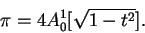Also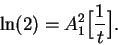My routines and calculations are given below. Here leftsum, rightsum and average are all procedures with four arguments, f,a,b, and n.

f is a function.

a and b are the endpoints of an interval.

n is the number of subintervals in a partition of [a,b].

The functions F and G are defined by F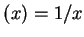and G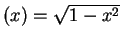. The command

             average(F,1.,2.,10000);

estimates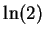by considering the regular partition of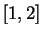into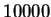equal subintervals. and the command
             4*average(G,0.,1.,2000);

estimates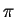by considering the regular partition of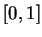into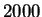equal subintervals.

> leftsum :=
>       (f,a,b,n) -> (b-a)/n*sum(f( a +((j-1)*(b-a))/n),j=1..n);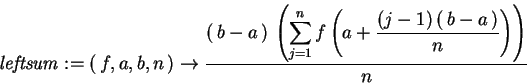> rightsum :=
>       (f,a,b,n) -> (b-a)/n*sum(f( a +(j*(b-a))/n),j=1..n);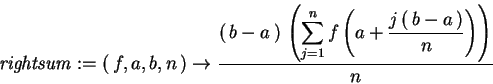> average :=
>       (f,a,b,n) -> (leftsum(f,a,b,n) + rightsum(f,a,b,n))/2;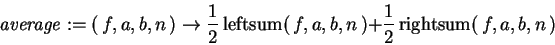> F := t -> 1/t;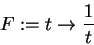> leftsum(F,1.,2.,10000);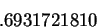> rightsum(F,1.,2.,10000);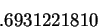> average(F,1.,2.,10000);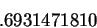> ln(2.);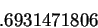> G := t -> sqrt(1-t^2);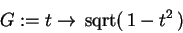> 4*leftsum(G,0.,1.,2000);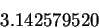> 4*rightsum(G,0.,1.,2000);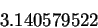> 4*average(G,0.,1.,2000);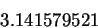> evalf(Pi);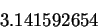Observe that in these examples, average yields much more accurate approximations than either leftsum or rightsum.Next: 6. Limits of Sequences Up: 5. Area Previous: 5.5 Brouncker's Formula For   Index
Ray Mayer 2007-09-07